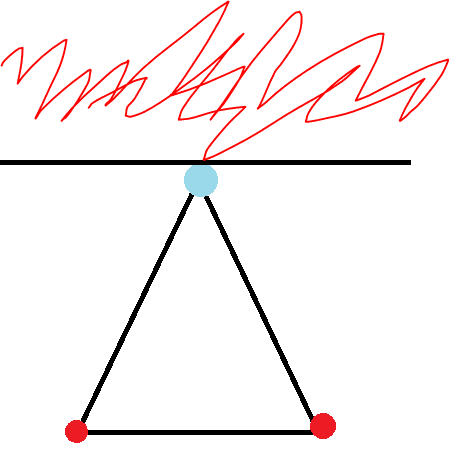# Three charges attached to the vertices of a triangle

LCSphysicist
Homework Statement:
Two positive ions and one negative ion are fixed at the vertices of
an equilateral triangle. Where can a fourth ion be placed, along the
symmetry axis of the setup, so that the force on it will be zero? Is
there more than one such place? You will need to solve something
numerically.
Relevant Equations:
Coulomb's Law would be enough.
Actually we find the two position in the axis very easily, but, what am trying to find is if exist such position (Being the charge of the ions equal) away from the symmetry axis, but i really don't want to try find it numerically, it would be a disaster.
The only conclusion i got is, if such position exist, it should be somewhere in the red region of the figure:The blue charge is negative
The red positive.

There is a way to prove if exist or not this position? Without the math would arise?

CPW
You will need to use math.

Solving a similar example problem, with the algebra and trig being performed on symbols (saving the numerical substitution for the last step), may provide you with the insight you need for this homework problem.

See this solution, and don't just read it. Instead work it out on paper.

•LCSphysicist
Homework Helper
Gold Member
2022 Award
I sketched the field lines. It seems to me there can only be the two saddle points.

•LCSphysicist
Mentor
Note that the question does not specify the sign of the "test" ion. It simply calls it "a fourth ion". It also doesn't ask for how many null force points exist, only if there is more than one.

Presumably, given the geometry of the setup, there must be at least one such point for a given test ion. So if there are two choices of charge polarity for the test ion, there must be at least two such points...

•hutchphd
Homework Helper
Gold Member
2022 Award
Note that the question does not specify the sign of the "test" ion. It simply calls it "a fourth ion". It also doesn't ask for how many null force points exist, only if there is more than one.

Presumably, given the geometry of the setup, there must be at least one such point for a given test ion. So if there are two choices of charge polarity for the test ion, there must be at least two such points...
I don't see how the polarity of the test ion matters. A zero field is a zero field.

Assuming all three charges have the same magnitude, there will will always be a saddle point in the potential near the midpoint between the two like charges, just a little further away than that from the third charge, and another on the far side of that third charge, roughly twice as far from the like charges as from the third charge.

For each of the combinations of of one charge and one saddle point, there is a unique field line connecting them. Using the diagram layout, there is a field line from the lower left charge to each saddle point, and a straight field line from each of those to the top charge. Together, these lines bound roughly a semicircle. Inside that, all field lines run from the lower left charge to the top charge. This leaves no possibility of another saddle point in that region.
Etc.

•CPW and hutchphd